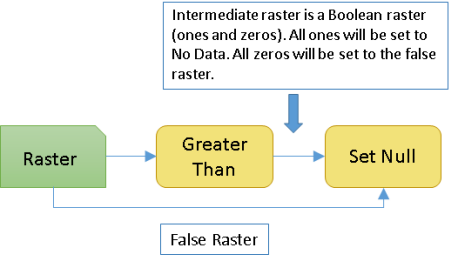# Set Null function

## Overview

Sets the identified pixels to NoData, based on the specified criteria. It returns NoData if a conditional evaluation is true (1) and returns the value specified in the False Raster if a conditional evaluation is false (0). This criteria is specified by the output of a logical math function, which will be the input Raster.

The Set Null function must be proceeded by a raster function within the Math: Logical category, such as the Greater Than function. The output from the logical function is a Boolean raster (values of 1 and 0). When using the Set Null function, all the values of 1 will be set to NoData, and all values of 0 will be set to the False Raster values.## Notes

If the evaluation is true, the pixel location on the output raster will be assigned NoData. If the evaluation is false, the output raster will be defined by the False Raster or constant value.

The condition is specified by a logical math raster function, which must be the input Raster.

If the False Raster (or constant value) contains floating-point values, the output raster will be floating point. If it contains all integer values, the output will be an integer raster.

## Parameters

Parameter nameDescription

Raster

The input raster that represents the Boolean result of a logical math raster function. The values will be either 1 (for true) or 0 (for false). It can be an integer or a floating-point raster.

False Raster

The input whose values will be used as the output pixel values if the condition is false. It can be an integer, a floating-point raster, or a constant value.

Extent Type

Choose which extent should be used in the output raster:

• First Of—Use the extent of the first input raster to determine the processing extent.

• Intersection Of—Use the extent of the overlapping pixels to determine the processing extent. This is the default.
• Union Of—Use the extent of all the rasters to determine the processing extent.
• Last Of—Use the extent of the last input raster to determine the processing extent.

Cellsize Type

Choose which cell size to use in the output raster. If all the input cell sizes are the same, all the options will yield the same results.

• First Of—Use the first cell size of the input rasters.
• Min Of—Use the smallest cell size of all the input rasters.
• Max Of—Use the largest cell size of all the input rasters. This is the default.
• Mean Of—Use the mean cell size of all the input rasters.
• Last Of—Use the last cell size of the input rasters.